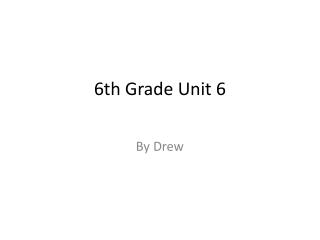DownloadDownload PresentationTélécharger la présentation- - - - - - - - - - - - - - - - - - - - - - - - - - - E N D - - - - - - - - - - - - - - - - - - - - - - - - - - -
##### Presentation Transcript

1. 6th Grade Unit 6 By Drew

2. Constant term • Some ting that stay the same like A+0=A no mater what a is it will be a added to 0 • Examples • Bx0=0 • U-0=U

3. Division of fractions property • When you dived fraction you flip the second fraction and multiply it • Examples • 2/4 dived by ¾ it would be 2/4 * 4/3 • 7/8 dived by 5/8 would be 7/8 * 8/5

4. Equation • An equation is when you use a letter to replace a number • Examples • W+9=12 W=3 • S-7=20 S=27

5. Equivalent equations • A Equivalent equations is seeing if two equations are equal • Examples • 1+3+a=a+3+1 • 2x4+b=4x2+b

6. Inequality • Inequality – not being equal • 2+2≠5 • 90/3≠21

7. Integers • A number that is not a decimal or a fraction such as … • 7 • 12 • 21

8. Multiplication property of -1 • Any positive number X -1 is that number – • Any negative number X -1 is positive number • 10 X (-1)=-10 • -10 X (-1)=10

9. Nested parentheses • Nested parentheses are parentheses are parentheses in parentheses • (2+2)X8)+4= • (3+3)X6)x3

10. Open sentence • Open sentence is a sentence that says something about math matrices • 5+5=10 • The opposite of 3 is -3

11. Opposite of a number • A opposite of a positive number is a negative number and other way a round • The opposite of 3 is -3 • The opposite of -17 is 17

12. Order of operations • PEMDAS (please excuse my dear aunt sally) Order in which you solve an equation. • Parentheses • Exponents • Multiplication and Division • Addition and Subtraction

13. Reciprocal • A reciprocal is a flipped fraction and a reciprocal times its fraction is always one • ½ 2/1 • 9/7 7/9 • ¾ X 4/3=1

14. Relation symbol • Symbols that show how numbers compare to each other. • 3>2 • 2x10=19+1

15. Repeating decimals • A Repeating decimals are decimals that go on for ever • 1/3=0.33333… • Pie 3.14…

16. Solution • An answer to a problem • 4+4=8 • 9X9=21

17. Solution set • The set containg all the solution • 2+2 the set would have 4 in it • 8X3 the set would have 24

18. Terminating decimals • A decimal number that has digits that don’t go on forever. • 0.23 • 0.75 • Rather then .66666…

19. Trial and error method • To find a solution, try one method. If it fails, try different methods until you find the answer. • Example: show your work.

20. Variable • A letter that takes the place of a number. • 3+x=4 • X=1 5x=15 X=3